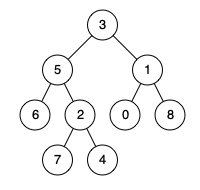0236. Lowest Common Ancestor of a Binary Tree

# 236. Lowest Common Ancestor of a Binary Tree#

## 题目 #

Given a binary tree, find the lowest common ancestor (LCA) of two given nodes in the tree.

According to the  definition of LCA on Wikipedia: “The lowest common ancestor is defined between two nodes p and q as the lowest node in T that has both p and q as descendants (where we allow a node to be a descendant of itself).”

Given the following binary tree: root = [3,5,1,6,2,0,8,null,null,7,4]Example 1:

``````Input: root = [3,5,1,6,2,0,8,null,null,7,4], p = 5, q = 1
Output: 3
Explanation: The LCA of nodes 5 and 1 is 3.
``````

Example 2:

``````Input: root = [3,5,1,6,2,0,8,null,null,7,4], p = 5, q = 4
Output: 5
Explanation: The LCA of nodes 5 and 4 is 5, since a node can be a descendant of itself according to the LCA definition.
``````

Note:

• All of the nodes’ values will be unique.
• p and q are different and both values will exist in the binary tree.

## 解题思路 #

• 这是一套经典的题目，寻找任意一个二叉树中两个结点的 LCA 最近公共祖先，考察递归

## 代码 #

``````
package leetcode

/**
* Definition for TreeNode.
* type TreeNode struct {
*     Val int
*     Left *ListNode
*     Right *ListNode
* }
*/
func lowestCommonAncestor236(root, p, q *TreeNode) *TreeNode {
if root == nil || root == q || root == p {
return root
}
left := lowestCommonAncestor236(root.Left, p, q)
right := lowestCommonAncestor236(root.Right, p, q)
if left != nil {
if right != nil {
return root
}
return left
}
return right
}

``````